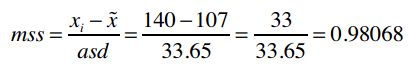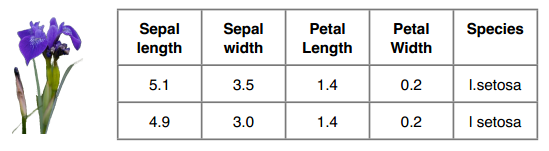## Python编码``````# 1
{'Asuka Termoto': ('Gymnastics', [54, 66]),
'Brittainey Raven': ('Basketball', [72, 162]), ...}
``````

``````# 2
[['Asuka Termoto', 'Gymnastics', 54, 66],
['Brittainey Raven', 'Basketball', 72, 162], ...]
``````

``````# 3
[('Gymnastics', [54, 66], ['Asuka Termoto']),
('Basketball', [72, 162], ['Brittainey Raven']), ...]
``````

``````class Classifier:

def __init__(self, filename):

self.medianAndDeviation = []

# 读取文件
f = open(filename)
f.close()
self.format = lines.strip().split('\t')
self.data = []
for line in lines[1:]:
fields = line.strip().split('\t')
ignore = []
vector = []
for i in range(len(fields)):
if self.format[i] == 'num':
vector.append(int(fields[i]))
elif self.format[i] == 'comment':
ignore.append(fields[i])
elif self.format[i] == 'class':
classification = fields[i]
self.data.append((classification, vector, ignore))
``````

``````>>> heights = [54, 72, 78, 49, 65, 63, 75, 67, 54]
>>> median = classifier.getMedian(heights)
>>> median
65
>>> asd = classifier.getAbsoluteStandardDeviation(heights, median)
>>> asd
8.0
``````

### 关于断言

``````def unitTest():
list1 = [54, 72, 78, 49, 65, 63, 75, 67, 54]
classifier = Classifier('athletesTrainingSet.txt')
m1 = classifier.getMedian(list1)
assert(round(m1, 3) == 65)
...
print("getMedian和getAbsoluteStandardDeviation均能正常工作")
``````

``````def getMedian(self, alist):
"""返回中位数"""

"""请在此处编写代码"""
return 0
``````

``````[54, 72, 78, 49, 65, 63, 75, 67, 54]
``````

assert（断言）表示函数的返回值应该是65。如果所有的单元测试都能通过，则报告以下信息：

``````getMedian和getAbsoluteStandardDeviation均能正常工作
``````

``````File "testMedianAndASD.py", line 78, in unitTest
assert(round(m1, 3) == 65)
AssertError
``````### 答案

``````def getMedian(self, alist):
"""返回中位数"""
if alist == []:
return []
blist = sorted(alist)
length = len(alist)
if length % 2 == 1:
# 列表有奇数个元素，返回中间的元素
return blist[int(((length + 1) / 2) -  1)]
else:
# 列表有偶数个元素，返回中间两个元素的均值
v1 = blist[int(length / 2)]
v2 = blist[(int(length / 2) - 1)]
return (v1 + v2) / 2.0

def getAbsoluteStandardDeviation(self, alist, median):
"""计算绝对偏差"""
sum = 0
for item in alist:
sum += abs(item - median)
return sum / len(alist)
``````

``````[('Gymnastics', [54, 66], ['Asuka Teramoto']),
('Gymnastics', [49, 90], ['Gabby Douglas']), ...]
``````

``````[('Gymnastics', [-1.93277, -1.21842], ['Asuka Teramoto']),
('Gymnastics', [-2.7731, -0.50520]),
('Track', [-0.08403, -0.23774], ['Helalia Johannes']),
('Track', [-0.42017, -0.02972], ['Irina Miketenko']), ...]
``````

``````# 获取向量的长度
self.vlen = len(self.data)
# 标准化
for i in range(self.vlen):
self.normalizeColumn(i)
``````

### 答案

``````def normalizeColumn(self, columnNumber):
"""标准化self.data中的第columnNumber列"""
# 将该列的所有值提取到一个列表中
col = [v[columnNumber] for v in self.data]
median = self.getMedian(col)
asd = self.getAbsoluteStandardDeviation(col, median)
#print("Median: %f   ASD = %f" % (median, asd))
self.medianAndDeviation.append((median, asd))
for v in self.data:
v[columnNumber] = (v[columnNumber] - median) / asd
``````

``````[(65.5, 5.95), (107.0, 33.65)]
````````````def normalizeVector(self, v):
"""我们已保存了每列的中位数和绝对偏差，现用它来标准化向量v"""
vector = list(v)
for i in range(len(vector)):
(median, asd) = self.medianAndDeviation[i]
vector[i] = (vector[i] - median) / asd
return vector
``````

``````classifier.classify([70, 140])
``````

``````def classify(self, itemVector):
"""预测itemVector的分类"""
return self.nearestNeighbor(self.normalizeVector(itemVector))
``````

### 答案

``````def manhattan(self, vector1, vector2):
"""计算曼哈顿距离"""
return sum(map(lambda v1, v2: abs(v1 - v2), vector1, vector2))

def nearestNeighbor(self, itemVector):
"""返回itemVector的近邻"""
return min([(self.manhattan(itemVector, item), item)
for item in self.data])
````````````-         Track  Aly Raisman  Gymnastics  62  115
...
+         Track  Xueqin Wang  Track 64  110
+         Track  Zhu Xiaolin  Track 67  123

80.00% correct
``````

### 鸢尾花数据集Ronald Fisher是一名伟大的科学家。他对统计学做出了革命性的改进，Richard Dawkins称他为“继达尔文后最伟大生物学家。”``````>>> test('irisTrainingSet.data', 'iristestSet.data')
93.33% correct
``````